8.5.5 Symmetric Case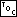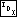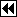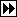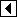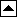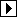Here's the code for the symmetric case.

```323. <Steps 3 and 4: Symmetric case in TAVL deletion 323> =
dir = q->tavl_link != y;
y->tavl_balance–;
if (y->tavl_balance == -1)   break;
else if (y->tavl_balance == -2)   {
struct tavl_node *x = y->tavl_link;
assert (x != NULL);
if (x->tavl_balance == +1)       {
<Rebalance for + balance factor after TAVL deletion in right subtree 324>
}     else       {

if (x->tavl_balance == 0)           {
<Rebalance for 0 balance factor after TAVL deletion in right subtree 325>
break;
}         else /* x->tavl_balance == -1 */           {
<Rebalance for - balance factor after TAVL deletion in right subtree 326>
}
}
}
```

This code is included in 318.

```324. <Rebalance for + balance factor after TAVL deletion in right subtree 324> =
struct tavl_node *w;

<Rebalance for + balance factor in TAVL insertion in left subtree 307>
```

This code is included in 323.

```325. <Rebalance for 0 balance factor after TAVL deletion in right subtree 325> =
x->tavl_balance = +1;
y->tavl_balance = -1;
```

This code is included in 323 and 444.

```326. <Rebalance for - balance factor after TAVL deletion in right subtree 326> =
if (x->tavl_tag == TAVL_CHILD)